# Solution assignment 03 Root equations

### Assignment 3

Solve: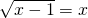### Solution

The following conditions have to be met: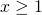and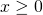thus:Squaring both sides yields: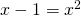This quadratic equation has a discriminant less than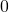: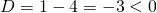and thus the equation has no solutions. This also holds for the original equation.